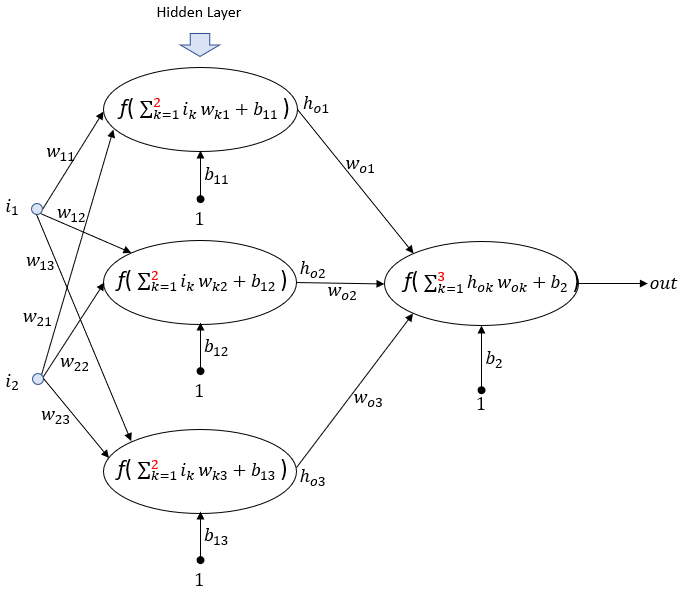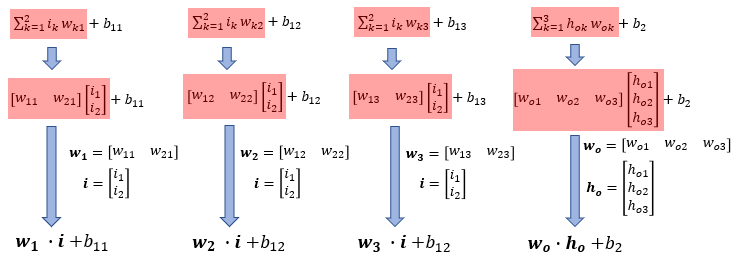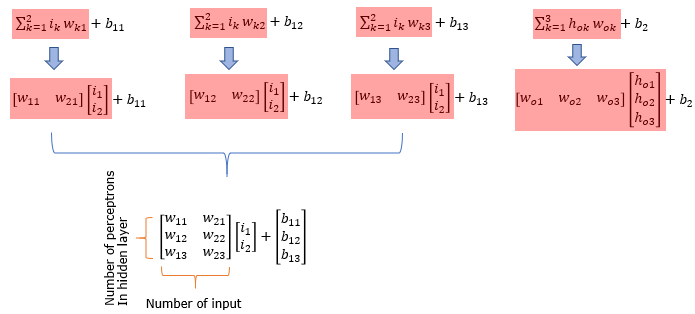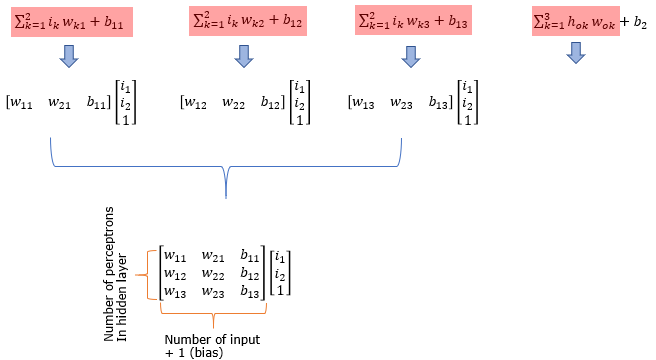Neural Network - Perceptron                                 Home : www.sharetechnote.com

Perceptron with 1 Hidden Layer

Assuming that you are now familiar with how single perceptron works and how it is represented in mathematical form (Read this page first before you start this page if you are not familiar with Single Perceptron).

There are only a limited cases that you can train to achieve what you want using single perceptron. For more complicated problems (like XOR problem), you would need to stack up multiple layers of perceptrons. One of the simplest examples of multi-layer perceptron would be as follows. In this example, you see a layer of two perceptrons are inserted between the input and output and this middle layer is called hidden layer. But the mathematical operation for each neuron (perceptron) is same.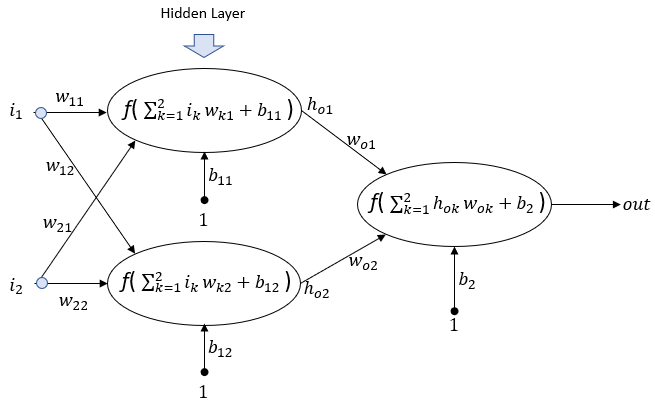Mathematical respresentation for each neuron (perceptron).

The Input to each perceptron is calculated in the same way as explained in single perceptron page. What I am want to do is to introduce a new mathematical expression.

The mathematical expressions on the top layer is same as you saw in single perceptron page. If you represent all the inputs and all the weight values for each perceptron into vector format, you can express the summation part into the inner product of two vectors as shown in the middle layer. Going further, if you represent each of these vectors to a corresponding simbols, you can simplify the summation part even further and get the expression as in the bottom layer. I strongly recommend you to get familiar with the vector notation as shown here since most of technical documents about neural network use these vector notation rather than using the summation symbol.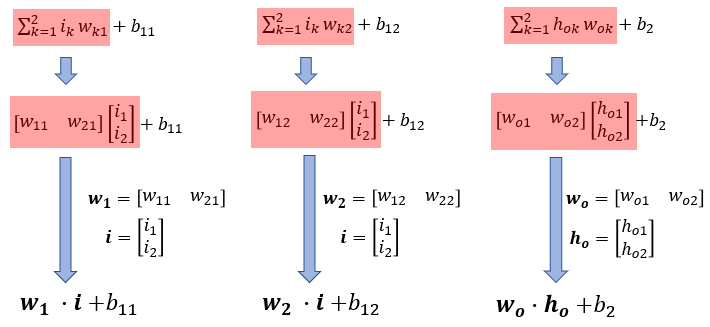Representing the neural network using this vector notation, the network in this example can be represented as follows.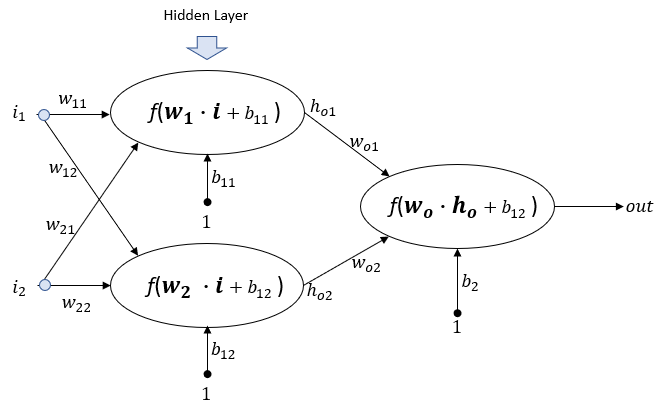In most machine learning (Neural Network) software, they tend to express each layer in a single matrix equation as shown below. I strongly recommend you to make practice of converting a network into this type of matrix equation.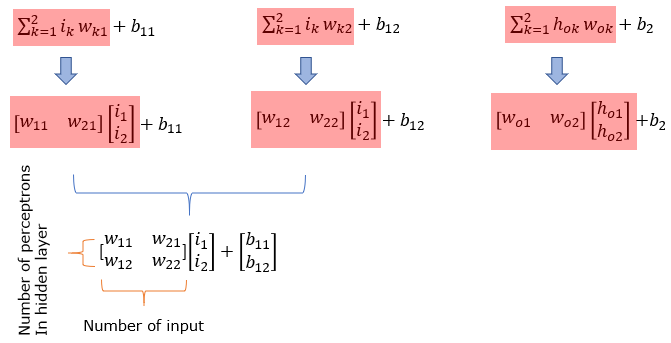You may simplify the math even further as shown below, but depending on the neural network software package this kind of simplification may or may not be allowed.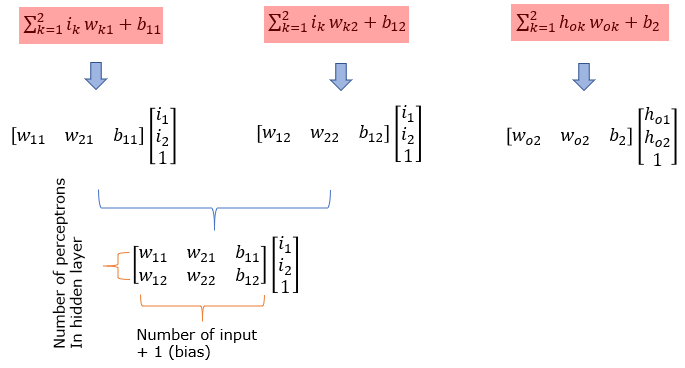Multiplayer Perceptron for XOR logic

One typical example of utilizing the simple network with one hidden layer which is made up of two perceptrons and one output perceptron is the neural network for XOR logic gate as described in the table below.

 in1 in2 out 1 1 0 1 0 1 0 1 1 0 0 0

More Math Practice on Representing a Network